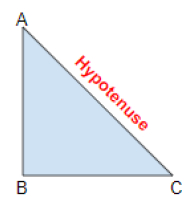# hypot( ), hypotf( ), hypotl( ) in C++

In this article we will be discussing the working, syntax and examples of hypot( ), hypotf( ), hypotl( ) function in C++.

## hypot( ) function

This function is used to compute the hypotenuse of a right angled triangle. This function returns the square root of sum of square of two variables. It is a function of <cmath> header file.

### What is hypotenuse?

Hypotenuse is the longest side of the right angle triangle. Below is the graphical representation of a hypotenuse in a right angle triangle.In above figure AC side of the triangle is a hypotenuse.

The formula to calculate hypotenuse is −

$$H = \sqrt{x^2+Y^2}$$

## Syntax

Data type hypot(data type X, data type Y);

## Parameters

The hypot( ) takes two or three parameters X, Y.

## Example

Inputs: X=3 Y=4
Output: 5
Input: X=12 Y=5
Output: 13

## Return value

The square root of (X2 + Y2)

## Approach can be followed

• First we initialize the two variables.

• Then we define the hypot( ) function.

• Then we print the square root.

By using above approach we can calculate the square root of sum of square of two variables. It is calculated by formula of h=sqrt(x2+y2).

## Example

// c++ program to demonstrate the working of hypot( ) function
#include<cmath.h>
#include<iostream.h>
Using namespace std;
int main( ){
// initialize the two values
int a=3, b=4, c;
cout<< “ A= ”<< a << “B= ” << b;
// define the hypot( ) function
c = hypot(a, b);
cout << “C= “ <<c<<endl;
double x, y, z;
x=12;
y=5;
cout<< “X=”<<x<< “Y=”<<y;
z = hypot(x, y);
cout<< “Z= “<<z;
return 0;
}

## Output

If we run the above code then it will generate the following output

OUTPUT - A=3 B=4
C= 5
OUTPUT - X=12 Y=5
Z=13

## hypotf( ) function

hypotf( ) function performs same task as hypot function. But difference is hypotf( ) function returns the float data type. And the parameter is also float type. It is a function of <cmath> header file.

### Syntax

float hypotf(float x);

### Example

Output – X= 9.34 Y=10.09
Z= 13.75
Output – X= 12.75 Y=5.56
Z= 13.90956

### Approach can be followed

• First we initialize the two variables in float data type.

• Then we define the hypotf( ) function.

• Then we print the square root.

By above we can calculate the square root.

## Example

// c++ program to demonstrate the working of hypotf( ) function
#include<iostream.h>
#include<cmath.h>
Using namespace std;
int main( ){
float x = 12.75, y = 5.56, z;
cout<< “X= “<<x<< “Y= “ <<y;
z = hypotf(x, y);
cout << “Z= “<<z;
return 0;
}

## Output

If we run the above code then it will generate the following output

OUTPUT – X= 12.75 Y=5.56
Z=13.90956
OUTPUT – X=9.34 Y=10.09
Z= 13.75

## hypotl( ) function

hypotl( ) function performs same task as the hypotl( ) function, but the difference is hypotl( ) function return the long double data type. And the parameter is also long double data type. It is function of <cmath > header file.

### Syntax

Long double hypotl( long double z)

### Example

Output – X= 9.34 Y=10.09
Z= 13.75
Output – X= 12.75 Y=5.56
Z= 13.90956

### Approach can be followed

• First we initialize the two variables in long double data type.

• Then we define the hypotl( ) function.

• Then we print the square root.

By above we can calculate the square root.

## Example

// c++ program to demonstrate the working of hypotl( ) function
#include<iostream.h>
#include<cmath.h>
Using namespace std;
int main( ){
long double x = 9.342553435, y = 10.0987456456, z;
cout<< “X= “<<x<< “Y= “ <<y;
z = hypotl(x, y);
cout<< “Z= “<<z;
return 0;
}

## Output

If we run the above code then it will generate the following output

OUTPUT – X= 9.3425453435 Y=10.0987456456
Z=13.7575
OUTPUT – X= 12.5854555 Y=5.125984
Z= 184.6694021107363